top of page
• Hackers Realm

# Titanic Dataset Analysis using Python (Kaggle) | Classification | Machine Learning Project Tutorial

Updated: Jun 5

Discover the fascinating world of Titanic dataset analysis using Python and Kaggle. This in-depth blog tutorial explores classification techniques and machine learning algorithms. Dive into data preprocessing, feature engineering, and model evaluation. Learn how to build and fine-tune classification models for predicting survival. Enhance your skills in Python programming, data analysis, and machine learning through this comprehensive project tutorial. Join us on this captivating journey into the Titanic dataset! #TitanicDataset #Python #Kaggle #Classification #MachineLearning #DataAnalysisTitanic Dataset Analysis - Classification

In this project tutorial, we are going to train the dataset using the train.csv that includes training and validation. Afterwards, we will use the trained model to predict the test dataset results and upload them into the Kaggle. We will perform basic level training without using hyperparameter tuning.

You can watch the video-based tutorial with step by step explanation down below

## Dataset Information

The data has been split into two groups:

• training set (train.csv)

• test set (test.csv)

The training set should be used to build your machine learning models. For the training set, we provide the outcome (also known as the “ground truth”) for each passenger. Your model will be based on “features” like passengersgender and class. You can also use feature engineering to create new features.

The test set should be used to see how well your model performs on unseen data. For the test set, we do not provide the ground truth for each passenger. It is your job to predict these outcomes. For each passenger in the test set, use the model you trained to predict whether or not they survived the sinking of the Titanic.

We also include gender_submission.csv, a set of predictions that assume all and only female passengers survive, as an example of what a submission file should look like.Variable Notes

• pclass: A proxy for socio-economic status (SES) 1st = Upper 2nd = Middle 3rd = Lower

• age: Age is fractional if less than 1. If the age is estimated, is it in the form of xx.5

• sibsp: The dataset defines family relations in this way...

• Sibling = brother, sister, stepbrother, stepsister

• Spouse = husband, wife (mistresses and fiancés were ignored)

• parch: The dataset defines family relations in this way...

• Parent = mother, father

• Child = daughter, son, stepdaughter, stepson

• Some children travelled only with a nanny, therefore parch=0 for them.

• The output class is survival, where we have to predict 0 (No) or 1 (Yes).

## Import modules

Let us import all the basic modules we will be needing for this project.

```import pandas as pd
import numpy as np
import seaborn as sns
import matplotlib.pyplot as plt
import warnings
warnings.filterwarnings('ignore')
%matplotlib inline```
• pandas - used to perform data manipulation and analysis

• numpy - used to perform a wide variety of mathematical operations on arrays

• matplotlib - used for data visualization and graphical plotting

• seaborn - built on top of matplotlib with similar functionalities

• %matplotlib - to enable the inline plotting.

• warnings - to manipulate warnings details

• filterwarnings('ignore') is to ignore the warnings thrown by the modules (gives clean results)

We will use Kaggle to load the data set.

```train = pd.read_csv('/kaggle/input/titanic/train.csv')Titanic Dataset
• We have to combine the train and test data. It will allow us to preprocess the data all at once.

```## statistical info
train.describe()```Statistical Information of Dataset
• We will fill the missing values using the range values (mean, minimum and maximum values).

```## datatype info
train.info()```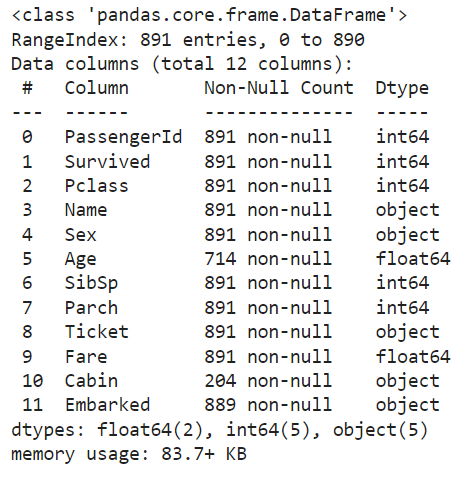Datatype Information
• We will convert the string values into integers later.

## Exploratory Data Analysis

Before preprocessing let us explore the categorical columns.

```## categorical attributes
sns.countplot(train['Survived'])```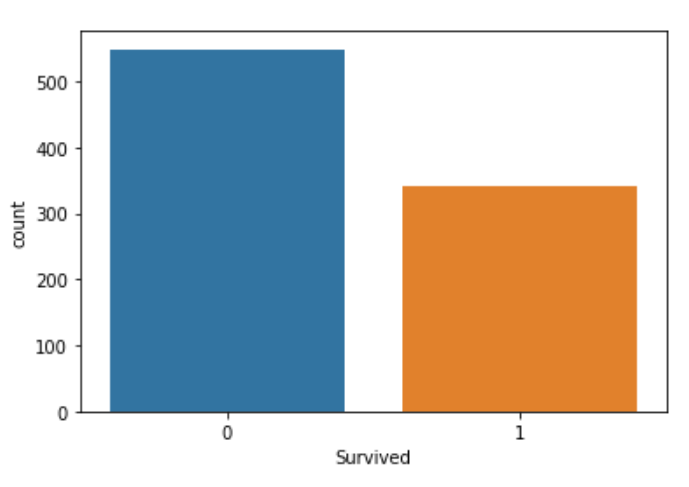Distribution of Survived
• The distribution of data is reasonable.

`sns.countplot(train['Pclass'])`Distribution of Pclass
• There is uneven distribution due to the 3rd class passengers.

`sns.countplot(train['Sex'])`Distribution of Sex
• We observe more males than females.

`sns.countplot(train['SibSp'])`Distribution of SibSP
• 0 indicates that the passenger is travelling solo.

`sns.countplot(train['Parch'])`Distribution of Parch

`sns.countplot(train['Embarked'])`Distribution of Embarked
• Embarked contains the boarding port/cities of passengers.

• There are three cities with S having the more number of values.

Let us explore the numerical columns.

```## numerical attributes
sns.distplot(train['Age'])```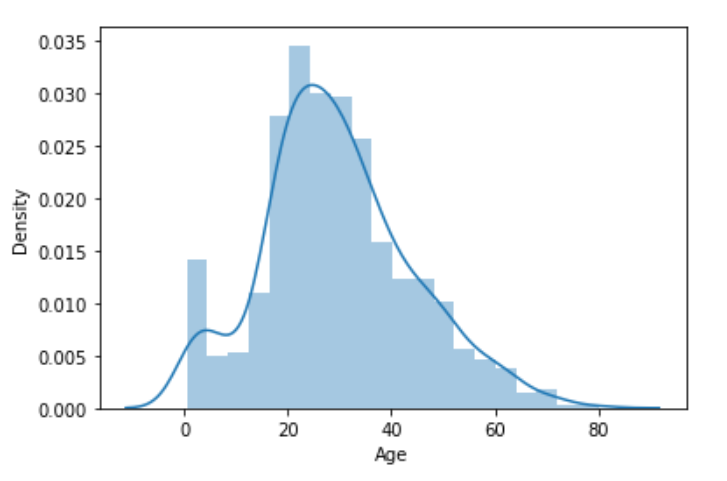Distribution of Age
• The graph shows a bell curve indicating a normal distribution.

`sns.distplot(train['Fare'])`Distribution of Fare
• We need to do preprocessing these data to convert the right-skewed curve into a normal distribution.

Let us compare ticket classes by creating a new graph using a pivot table.

```class_fare = train.pivot_table(index='Pclass', values='Fare')
class_fare.plot(kind='bar')
plt.xlabel('Pclass')
plt.ylabel('Avg. Fare')
plt.xticks(rotation=0)
plt.show()```Bar Plot of Pclass and Average Fare
• It will help us to make an assumption on fares and the ticket class.

Let's compare Pclass by creating a new graph using a pivot table.

```class_fare = train.pivot_table(index='Pclass', values='Fare', aggfunc=np.sum)
class_fare.plot(kind='bar')
plt.xlabel('Pclass')
plt.ylabel('Total Fare')
plt.xticks(rotation=0)
plt.show()```Bar Plot of Pclass and Total Fare
• All these visualizations help in understanding the variation of the dataset depending on the attributes.

Let us display the difference between 'Pclass' and 'Survived' with the help of a barplot.

`sns.barplot(data=train, x='Pclass', y='Fare', hue='Survived')`• This plot has a comparison of survived passengers depending on the ticket fare and passenger class.

Let's change the horizontal and vertical axis of the graph.

`sns.barplot(data=train, x='Survived', y='Fare', hue='Pclass')`• Similar to the previous graph, it shows the comparison of survived passengers.

## Data Preprocessing

We now combine the train and test datasets.

```train_len = len(train)
# combine two dataframes
df = pd.concat([train, test], axis=0)
df = df.reset_index(drop=True)• train_len is for the length of train data.

• axis=0 means it will concatenate in respect of row.

• axis=1 means it will concatenate in respect of columns.

• df.head() displays the first five rows from the data frame.

`df.tail()`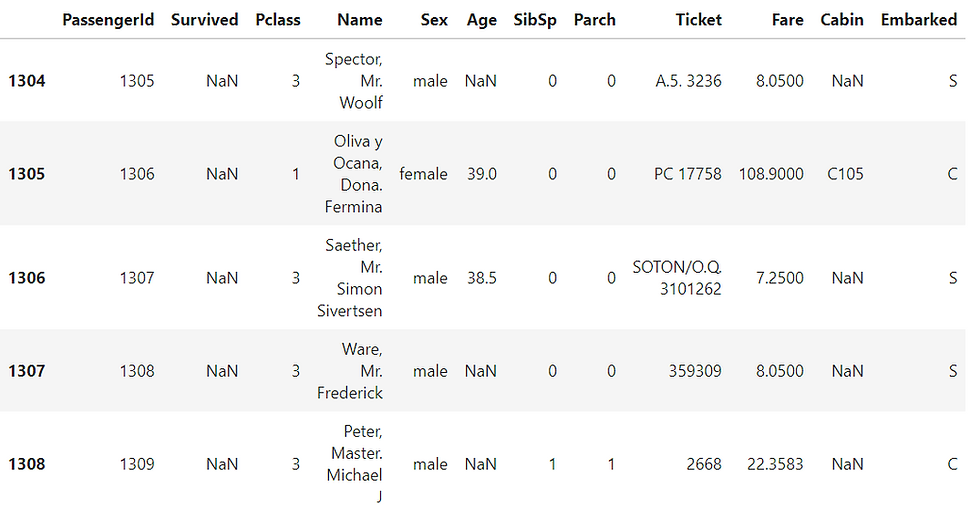• df.tail() displays the last five rows from the data frame.

Let us check for NULL values in the dataset.

```## find the null values
df.isnull().sum()```Count of NULL Values
• Survived attributes NULL values are for the test data. Hence, we can avoid its NULL values.

• Since the cabin has more than a thousand NULL values, we need to drop the column.

• We will fill the missing values for other columns that show null values using the mean.

Let us remove column 'Cabin'.

```# drop or delete the column
df = df.drop(columns=['Cabin'], axis=1)```

The mean value of column 'Age'.

`df['Age'].mean()`

29.88

We will use the mean values to fill the missing values for 'Age' and 'Fare'.

```# fill missing values using mean of the numerical column
df['Age'] = df['Age'].fillna(df['Age'].mean())
df['Fare'] = df['Fare'].fillna(df['Fare'].mean())```

The mean value of column 'Embarked'.

`df['Embarked'].mode()`

'S'

• The mode values return an dataframe, so we will use subscript to get the value.

Similarly, we will use the mode value to fill the missing values for 'Embarked'.

```# fill missing values using mode of the categorical column
df['Embarked'] = df['Embarked'].fillna(df['Embarked'].mode())```
• We use mode to fill the missing values of the categorial column.

## Log transformation for Normal data distribution

We have to normalize the column 'Fare'.

`sns.distplot(df['Fare'])``df['Fare'] = np.log(df['Fare']+1)`
• If the 'fare' has a '0' value then it will result in an error.

• To resolve this issue we have to add +1 in log transformation.

`sns.distplot(df['Fare'])`• It is not a complete normal distribution, but we can manage with this curve.

## Correlation Matrix

A correlation matrix is a table showing correlation coefficients between variables. Each cell in the table shows the correlation between two variables. The value is in the range of -1 to 1. If two variables have a high correlation, we can neglect one variable from those two.

```corr = df.corr()
plt.figure(figsize=(15, 9))
sns.heatmap(corr, annot=True, cmap='coolwarm')```Correlation Matrix
• The 'Fare' shows a negative correlation with Pclass.

• Additionally, Fare has some level of correlation with all classes. Hence, the Fare column is an essential attribute for this project.

Let us display the dataset again.

`df.head()`Now, we will remove a few unnecessary columns.

```## drop unnecessary columns
df = df.drop(columns=['Name', 'Ticket'], axis=1)## Label Encoding

Label Encoding refers to converting the labels into the numeric form and converting them into the machine-readable form. We will convert the column 'Sex' and 'Embarked'.

```from sklearn.preprocessing import LabelEncoder
cols = ['Sex', 'Embarked']
le = LabelEncoder()

for col in cols:
df[col] = le.fit_transform(df[col])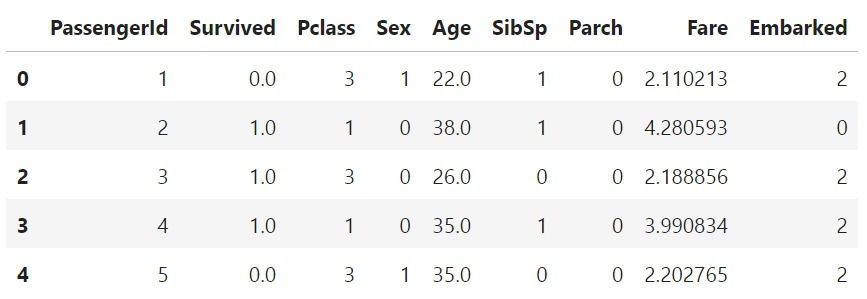• In column 'Sex', the male is converted to '1' and the female is converted to '0'.

• Likewise in 'Embarked' the cities are assigned some defined number.

## Train-Test Split

Let's split the dataset for train and test data.

```train = df.iloc[:train_len, :]
test = df.iloc[train_len:, :]```
`train.head()`• We have all the data required for training and testing.

`test.head()`• Survived columns show null value.

• We need to drop the column 'PassengerId' and 'Survived'.

```# input split
X = train.drop(columns=['PassengerId', 'Survived'], axis=1)
y = train['Survived']```
`X.head()`• We will use these input attributes for model training.

## Model Training

Now the preprocessing has been done, let's perform the model training and testing.

• If you train and test the dataset completely, the results will be inaccurate. Hence, we will use 'train_test_split'.

• We will add random_state with the attribute 42 to get the same split upon re-running.

• If you don't specify a random state, it will randomly split the data upon re-running giving inconsistent results.

```from sklearn.model_selection import train_test_split, cross_val_score
# classify column
def classify(model):
x_train, x_test, y_train, y_test = train_test_split(X, y, test_size=0.25, random_state=42)
model.fit(x_train, y_train)
print('Accuracy:', model.score(x_test, y_test))

score = cross_val_score(model, X, y, cv=5)
print('CV Score:', np.mean(score))```
• X contains input attributes and y contains the output attribute.

• We use cross val score() for better validation of the model.

• Here, cv=5 means that the cross-validation will split the data into 5 parts.

• np.abs() will convert the negative score to positive and np.mean() will give the average value of 5 scores.

• Let's train our data with different models.

Logistic Regression:

```from sklearn.linear_model import LogisticRegression
model = LogisticRegression()
classify(model)```
• Model report: Accuracy = 0.8071 CV Score = Nan

Decision Tree:

```from sklearn.tree import DecisionTreeClassifier
model = DecisionTreeClassifier()
classify(model)```
• Model report: Accuracy = 0.7309 CV Score = 0.7650

Random Forest:

```from sklearn.ensemble import RandomForestClassifier
model = RandomForestClassifier()
classify(model)```
• Model report: Accuracy = 0.7892 CV Score = 0.7654

Extra Trees:

```from sklearn.ensemble import ExtraTreesClassifier
model = ExtraTreesClassifier()
classify(model)```
• Model report: Accuracy = 0.7937 CV Score = 0.7923

XGBoost:

```from xgboost import XGBClassifier
model = XGBClassifier()
classify(model)```
• Model report: Accuracy = 0.7892 CV Score = 0.8125

LightGBM:

```from lightgbm import LGBMClassifier
model = LGBMClassifier()
classify(model)```
• Model report: Accuracy = 0.8116 CV Score = 0.8238

CatBoost:

```from catboost import CatBoostClassifier
model = CatBoostClassifier(verbose=0)
classify(model)```
• Model report: Accuracy = 0.8296 CV Score = 0.8226

Among all the models, LightGBM shows the highest CV score.

## Complete Model Training with Full Train Data

Before submitting our model, we have to train it with the full data.

```model = LGBMClassifier()
model.fit(X, y)```

Let's print the test data again.

`test.head()`Now, we have to drop unnecessary columns from the test data.

```# input split for test data
X_test = test.drop(columns=['PassengerId', 'Survived'], axis=1)```
`X_test.head()`• As a result, we have training data similar to the input attributes.

We will check the prediction result in the next process.

```pred = model.predict(X_test)
pred```
• The predicted data will be in the form of an array.

• The predicted values will be in float format.

• We have to create a new data frame to store this predicted data.

## Test Submission

In the last step of the project, we will use the submission template to submit our predicted results. We have to submit the predicted data in PassengerId and Survived column.

```sub = pd.read_csv('/kaggle/input/titanic/gender_submission.csv')`sub.info()`• The predicted values are in the float format.

• Let's change it into integers before submitting the data.

```sub['Survived'] = pred
sub['Survived'] = sub['Survived'].astype('int')```
`sub.info()`• Now both the attributes are of integer datatype.

`sub.head()``sub.to_csv('submission.csv', index=False)`
• index=false will drop the index and save the two columns.

• We can submit this file to the Kaggle and check the results.

## Final Thoughts

• You can improve your model performance for better accuracy.

• To achieve higher accuracy, you can perform hyperparameter tuning or create new attributes using existing ones.

• In addition to this basic feature, you can also incorporate other advanced techniques to improve the accuracy of your model.

In this project tutorial, we have discussed the baseline codes for Titanic Dataset Analysis. We also used different models to achieve the best accuracy for our prediction. Finally, we submitted our predicted result to the Kaggle project folder.

Get the project notebook from here